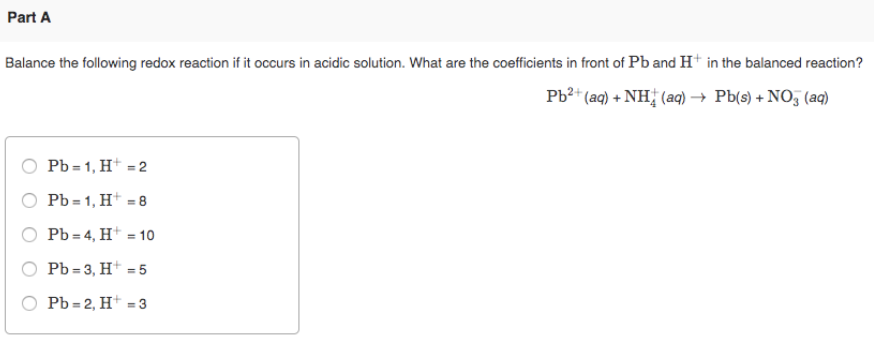# Balanced the following redox reaction if it occurs in acidic solution. What are the coefficients in front of Pb and H^+ in the balanced reaction? Pb^2+(aq) + NH4^+(aq) → Pb(s) + NO3^-(aq) a) Pb = 1, H^+ = 2 b) Pb = 1, H^+ = 8 c) Pb = 4, H^+ = 10 d) Pb = 3, H^+ = 5 Pb = 2, H^+ = 3